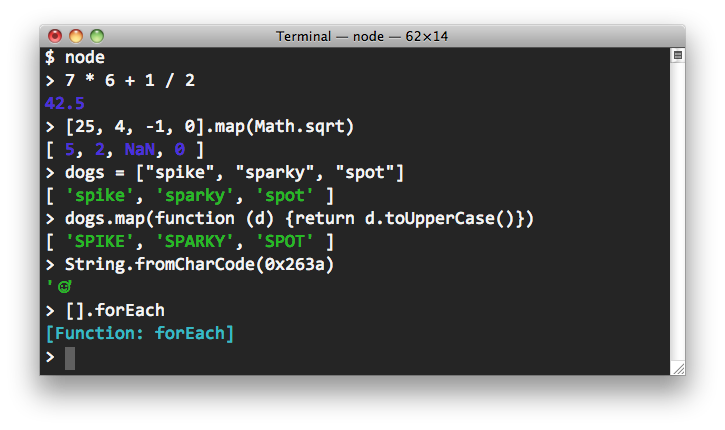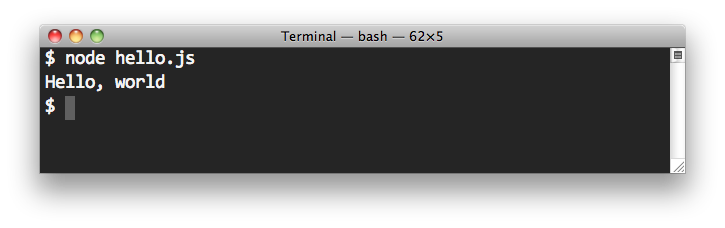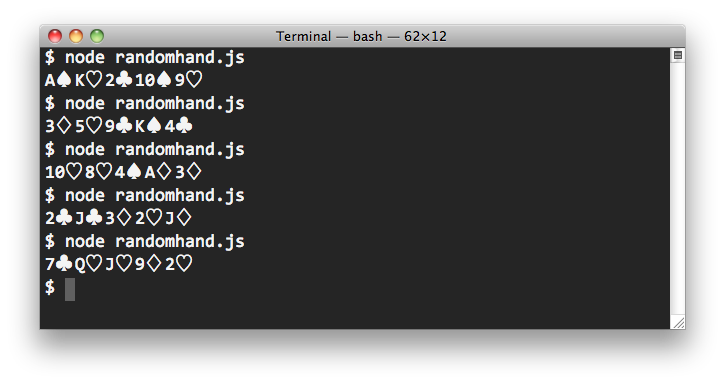# Command Line JavaScript

Our latest book covers client-side JavaScript — scripts that run in web browsers (both desktop and mobile). JavaScript applications do not have to run in a browser, though; you can create applications that run right from your system's Terminal, or command line. The most common way to build and run such scripts is with Node.js, or node for short.

## Installing Node.jsNode.js is a very popular platform that is used to build fast, scalable network applications, typically servers. Here we're interested in simply using node for running JavaScript applications outside of the browser.

First, download and install node. How you do this depends on the kind of machine and operating system you have. Fortunately there are installation instructions on node's github wiki for installing either with a package manager or installer, or from source.

Once you have installed node, you can test your installation by entering the following on the command line:

`node -v`

This will respond with the version number of the version you just installed.

## The Node REPL (Shell)

If you enter `node` on the command line with no arguments, you'll be in the Read-Eval-Print-Loop, or REPL for short, otherwise known as a shell. Here you can interactively enter JavaScript expressions and have them immediately evaluated (just as in the shell at squarefree.com we mentioned in the book).## A First JavaScript Command Line Application

The shell is great for practice, but we want to write programs, store them in files, and execute them on demand. Let's start with Hello, world. Create the file hello.js containing just one line:

```console.log('Hello, world');
```

Node's built-in `console` object allows you to write to the terminal. Technically, it allows writing to your system's standard output and standard error streams, but we'll get to that later. Running your program is easy; invoke `node` on the file containing your program:## More Examples

Here are a couple simple applications of the kind that seem to appear in introductory programming lessons. The first displays powers of two:

```/*
* A command line application for generating powers of two
* from 2^0 through 2^32.
*/
for (var x = 1, i = 0; i <= 32; i += 1) {
console.log(x);
x += x;
}
```

Save this program in the file powers.js and enter `node powers.js` in your terminal to run the program.

You can make use of `console.log`'s formatting abilities, too:

```/*
* A command line application for generating powers of two
* from 2^0 through 2^32.
*/
for (var x = 1, i = 0; i <= 32; i += 1) {
console.log('2^%d = %d', i, x);
x += x;
}
```

The first argument to `console.log` is a string which may contain format specifiers, which are used to format the additional arguments. The specifier `%d` means "as a number" so the first few lines of output will be:

```2^0 = 1
2^1 = 2
2^2 = 4
2^3 = 8
2^4 = 16
2^5 = 32
```

And here is a simple program to log the first few rows of the famous Pascal's Triangle:

```/*
* An application that writes the first 16 rows of Pascal's Triangle.
*/

var LIMIT = 16;

// The triangle will be an array of rows.  Each row will be an array.
var triangle = [];

// Fill in the triangle using the well-known formula.
for (var row = 0; row < LIMIT; row += 1) {
triangle.push([]);
for (var column = 0; column <= row; column += 1) {
if (column === 0 || column === row) {
triangle[row][column] = 1;
} else {
triangle[row][column] = triangle[row-1][column-1] + triangle[row-1][column];
}
}
}

// Display the data, row by row.
for (var row = 0; row < triangle.length; row += 1) {
console.log(triangle[row].join(' '));
}
```

The output begins:

```1
1 1
1 2 1
1 3 3 1
1 4 6 4 1
1 5 10 10 5 1
1 6 15 20 15 6 1
```

And here is a program that generates a random five-card poker hand every time it is run:

```/*
* An application that displays a random 5-card poker hand, by "dealing out"
* five random cards from a deck.  This is a very sloppy script that we will
* refactor later to use modules.
*/

// Create a deck of cards sorted by suit then rank.  The first card (at index
// 0) is the ace of spades (A♠), and the last (at index #51) is the king of
// clubs (K♣).
var deck = [];
"♠♡♢♣".split("").forEach(function (suit) {
"A 2 3 4 5 6 7 8 9 10 J Q K".split(" ").forEach(function (rank) {
deck.push(rank + suit);
});
});

// Create a hand by successively removing a random card from the deck
// and adding it to the hand.
var hand = [];
for (var i = 0; i < 5; i += 1) {
hand.push(deck.splice(Math.floor(Math.random() * deck.length), 1));
}

// Display the hand.
console.log(hand.join(" "));
```

Here are a few sample runs:## Command Line Arguments

Programs launched from the command line need to access their arguments, of course. In node, the arguments are placed in the array `process.argv`. For example, if we enter the following:

```node calendar.js --year 2020 --month 7
```

then `process.argv` will contain the array ```['node', 'calendar.js', '--year', '2020', '--month', '7']```. The first argument to your program will be at `process.argv`.

```/*
* A command line script to write the average of its two
* command line arguments, which will be treated as numbers.
* Example:
*
*     node average.js 84 22
*/
var x = +process.argv;
var y = +process.argv;
console.log((x + y) / 2);
```

The argument positioning remains the same even if you wish to make use of the shebang at the top of your script. On Unix-like systems, the first line of a script can name the interpreter that is to run the script, so your command line need not supply it. For example, if you have installed node in the directory `/usr/local/bin`, then you can write the following program in the file named average (not average.js), allowing you to invoke the program quite cleanly:

```#! /usr/local/bin/node

/*
* A command line script to write the average of its two
* command line arguments, which will be treated as numbers.
* Example:
*
*     average 84 22
*/
var x = +process.argv;
var y = +process.argv;
console.log((x + y) / 2);
```

If your script takes more than one or two commandline arguments, we strongly urge you to design your arguments to take named values (sometimes called options) and use a command line option parser. A large number of option parsers are available for node; pick any one you like.

## Reading and Writing Files

JavaScript applications that run in the browser are necessarily limited; for example, they cannot open and read files on your machine. If they were permitted to do so, you might (unintentionally, perhaps) navigate to a malicious site hosting a script that searched your file system for personal information and sent back whatever it found to its originating server. And, of course, browser-based scripts cannot write to your file system, either. You can probably guess why not.

Node, however, lets you read and write files. You can process files synchronously or asynchronously; you can process them in chunks or all-at-once. We'll give just one simple example.

```/*
* This script reads a file and produces a new file like the original with all
* the r's and l's replaced with w's.  The input file is assumed to be encoded
* using UTF-8; the output file will also be UTF-8 encoded.  The new file's name
* is formed from the input file name by appending a '.w'.
*
* For example, if the file 'greeting.txt' contained the text
*
*   Hello, how are you today?
*
* The executing
*
*   node fuddify.js greeting.txt
*
* will produce a new file named 'greeting.text.w' containing
*
*   Hewwo, how awe you today?
*/
var fs = require('fs');

if (process.argv.length !== 3) {
console.error('Exactly one argument required');
process.exit(1);
}

var input = process.argv;
var output = input + '.w';

// Read the entire file asynchronously, with a callback to replace the r's and l's
// with w's then write the result to the new file.
fs.readFile(input, 'utf-8', function (err, text) {
if (err) throw err;
var fuddified = text.replace(/[rl]/g, 'w').replace(/[RL]/g, 'W')
fs.writeFile(output, fuddified, function (err) {
if (err) throw err;
});
});
```

## What to Read Next

So, that was a pretty quick introduction to using node to write command line scripts, but we've barely scratched the surface of what node is and what it can do. You'll want to visit node's home page, watch Ryan Dahl's introductory video and read the documentation. If you are reading this current document because you are simply interested in JavaScript beyond the browser, there are a couple more pages in this series. See The CommonJS Module System and Server-Side JavaScript.